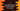# C example program to add numbers to an array### Example program to add numbers to an array using C :

In this example program, we will learn how to add numbers to an array in C programming language. What is an Array ? An array is a collection of items of same type. Arrays can be of one dimensional or multi dimensional. We will write one different post on ‘multidimension array’ . In this example, we are going to use single dimensional array. You can think it as like a list.

### Explanation of the problem :

This is a simple ‘array’ program in ‘c’.

• We will ask the user to enter how many numbers he/she wants to enter .
• Then, We will read the count and create one array as equal length of the count. i.e. if the user enters 5, we will create one array of size 5 or if enters 6, we will create one array of size 6.
• After that, we will run one for loop to read each numbers for that array and insert it into the array. This loop will run n number of times , where n is the size of the array.
• Finally, we will run one more for loop and print out all the numbers stored in the array. Let’s take a look into the program :

### C program :

``````#include <stdio.h>

int main()
{
//1
int i, total;

//2
printf("Enter total no of elements : ");
scanf("%d", &total);

//3
int myArray[total];

//4
for (i = 0; i < total; i++)
{
printf("Enter no %d : ", i + 1);
scanf("%d", &myArray[i]);
}

//5
printf("You have entered : ");
for (i = 0; i < total; i++)
{
printf("%d ", myArray[i]);
}
}``````

### Explanation :

Check the commented numbers in the above program . These numbers indicates the below step name .

1. Create two integer variables : i and total.
2. Read the total number of elements for the array and store it in variable total.
3. Create one array myArray and the size of this array is equal to the total number of elements i.e. same as total.
4. Use one for loop to read all numbers to store in myArray. Read the number and store it in the array using scanf.
5. Run one more for loop to print out the numbers entered by the user using printf.

### Sample Output :

``````Enter total no of elements : 5
Enter no 1 : 1
Enter no 2 : 2
Enter no 3 : 3
Enter no 4 : 4
Enter no 5 : 5
You have entered : 1 2 3 4 5

Enter total no of elements : 6
Enter no 1 : 1
Enter no 2 : 3
Enter no 3 : 4
Enter no 4 : 6
Enter no 5 : 9
Enter no 6 : 20
You have entered : 1 3 4 6 9 20

Enter total no of elements : 1
Enter no 1 : 2
You have entered : 2``````